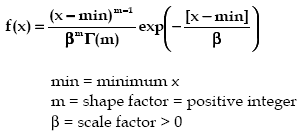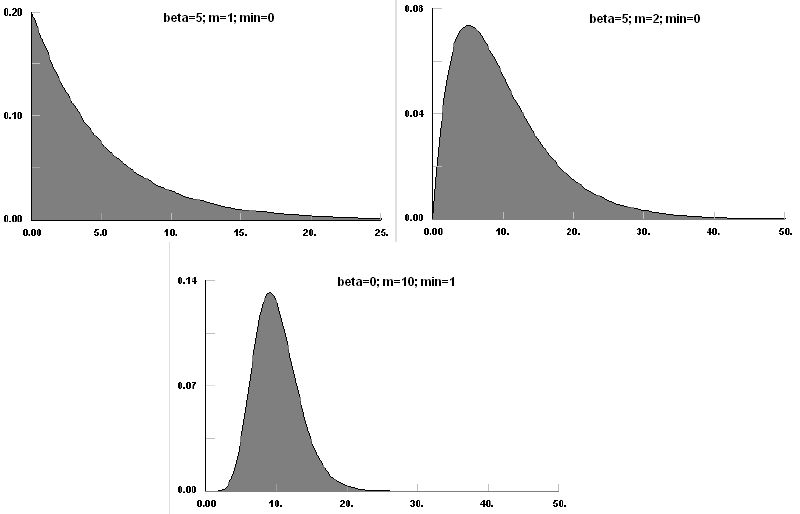AnyLogic

# erlangThe Erlang distribution is a continuous distribution bounded on the lower side. It is a special case of the Gamma distribution where the parameter, m, is restricted to a positive integer. As such, the Erlang distribution has no region where F(x) tends to infinity at the minimum value of x [m > 1], but does have a special case at m=1, where it reduces to the Exponential distribution.

The Erlang distribution has been used extensively in reliability and in queuing theory, thus in discrete event simulation, because it can be viewed as the sum of m exponentially distributed random variables, each with mean beta.

#### Examples### erlang(double beta, int m, double min)

Description
Generates a sample of the Erlang distribution.
Parameters
Name Type of value Description
beta double The scale factor > 0.
m int The shape factor (positive integer).
min double The minimum x value.
Result
Type Description
double The generated sample.

### erlang(double beta, int m)

Description
Generates a sample of the Erlang distribution with min set to 0. Is equivalent to erlang(beta, m, 0).
Parameters
Name Type of value Description
beta double The scale factor > 0.
m int The shape factor (positive integer).
Result
Type Description
double The generated sample.

### erlang(double beta, int m, double min, java.util.Random r)

Description
Generates a sample of the Erlang distribution using the specified random number generator.
Parameters
Name Type of value Description
beta double The scale factor > 0.
m int The shape factor (positive integer).
min double The minimum x value.
r java.util.Random The random number generator.
Result
Type Description
double the generated sample

This document includes content from the “Stat::Fit User’s Manual”. Copyright 2016 Geer Mountain Software Corp.